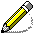Welcome to Klara Grodzinsky's Homepage!

In the Spring 2022 semester, I am teaching:

• MATH 1552, Integral Calculus

• CETL 2000/8000, TA Development Seminar

Students, please visit Canvas for course syllabi and information.

Semester classes I have taught at Georgia Tech include Math 1113 (Precalculus), Math 1501 (Calculus I), Math 1502 (Calculus II), Math 1522 (Introduction to Linear Algebra), Math 1550 (Differential Calculus), Math 1552 (Integral Calculus), Math 1553 (Introduction to Linear Algebra), Math 1711 (Finite Mathematics), Math 1712 (Survey of the Calculus), and Math 3012 (Applied Combinatorics).

Need extra help? Visit the Tutoring Center in Clough Commons. This site lists available tutoring around campus.

Looking for old tests and solutions to this semester's tests? You can find them on Canvas or on the Library Electronics Reserves page.

Last updated on December 18, 2020.